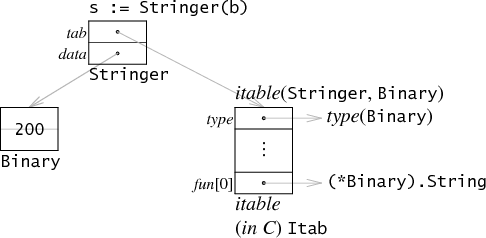Reflect -- static, checked at compile time, dynamic when asked for

Go语言是静态类型，所有数据的类型在编译器都是明确的，规定好了的。

``````type MyInt int

var i int
var j MyInt
``````

``````var r io.Reader
r = os.Stdin
r = new(bytes.Buffer)
// and so on
``````

interface{} 画外音：我就是一个什么接口也不要实现的接口类型，是不是显得很拉风？

``````type Stringer interface {
String() string
}

type Binary uint64

func (i Binary) String() string {
return strconv.Uitob64(i.Get(), 2)
}

func (i Binary) Get() uint64 {
return uint64(i)
}
``````Interface就存两个指针，一个是指向Binary的，另一个指向的是iTable（interface Table），

binary的Get在这里面就没有了。

``````package main

import (
"fmt"
"reflect"
)

func main() {
var x float64 = 3.4
fmt.Println("type:", reflect.TypeOf(x))
}
``````

// TypeOf returns the reflection Type of the value in the interface{}. func TypeOf(i interface{}) Type 首先x会存储在空的interface里面，也就是根据之前那张图来存储。

``````var x float64 = 3.4
fmt.Println("value:", reflect.ValueOf(x))
``````

valueOf 获得的是复制，对于它的修改对原来的数据是没有影响的。如果想要修改原值，就要用指针。再用p.Elem就能获得可以修改的对象了。

``````type T struct {
A int
B string
}
t := T{23, "skidoo"}
s := reflect.ValueOf(&t).Elem()
typeOfT := s.Type()
for i := 0; i < s.NumField(); i++ {
f := s.Field(i)
fmt.Printf("%d: %s %s = %v\n", i,
typeOfT.Field(i).Name, f.Type(), f.Interface())
}
``````

``````package main

import (
"fmt"
"reflect"
)

func main() {
// swap is the implementation passed to MakeFunc.
// It must work in terms of reflect.Values so that it is possible
// to write code without knowing beforehand what the types
// will be.
swap := func(in []reflect.Value) []reflect.Value {
return []reflect.Value{in, in}
}

// makeSwap expects fptr to be a pointer to a nil function.
// It sets that pointer to a new function created with MakeFunc.
// When the function is invoked, reflect turns the arguments
// into Values, calls swap, and then turns swap's result slice
// into the values returned by the new function.
makeSwap := func(fptr interface{}) {
// fptr is a pointer to a function.
// Obtain the function value itself (likely nil) as a reflect.Value
// so that we can query its type and then set the value.
fn := reflect.ValueOf(fptr).Elem()

// Make a function of the right type.
v := reflect.MakeFunc(fn.Type(), swap)

// Assign it to the value fn represents.
fn.Set(v)
}

// Make and call a swap function for ints.
var intSwap func(int, int) (int, int)
makeSwap(&intSwap)
fmt.Println(intSwap(0, 1))

// Make and call a swap function for float64s.
var floatSwap func(float64, float64) (float64, float64)
makeSwap(&floatSwap)
fmt.Println(floatSwap(2.72, 3.14))

}
``````

http://blog.golang.org/laws-of-reflection

http://research.swtch.com/interfaces